Next: 4.1.2 Assembly Up: 4.1 The Finite Element Previous: 4.1 The Finite Element

## 4.1.1 Galerkin's Method

Multiplying (4.1) by a function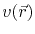, which is called test or trial function, and integrating over the simulation domain gives the variational formulation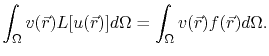(4.2)

Using the notation(4.3)

(4.2) can be written as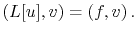(4.4)

In order to obtain the corresponding discrete problem, the simulation domain, , is divided in a set of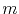elements,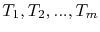, which do not overlap, i.e.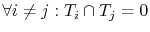. The mesh obtained by such a domain discretization is represented by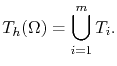(4.5)

Further, one defines a set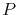of grid points, also called nodes, with each point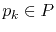being described by a unique global index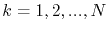, where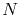is the total number of grid points in the mesh.

The approximate solution,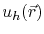, for the unknown function,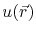, is given by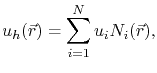(4.6)

where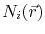are the so-called basis (or shape) functions. The approximate solution of (4.4) is determined by the coefficients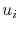, which represent the value of the unknown function at the node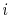. At the node, where the point is given by the coordinates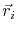, the basis functions must satisfy the condition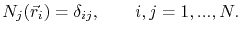(4.7)

Typically, the basis functions are chosen to be low order polynomials.

Substituting (4.6) in (4.4), and choosing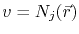one obtains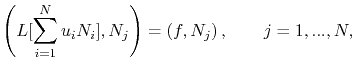(4.8)

and since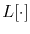is a linear operator and the coefficientsare constants one can write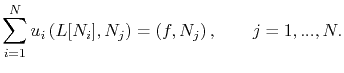(4.9)

Equation (4.9) is, in fact, a linear system ofequations withunknowns,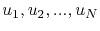. Thus, it can be written in matrix notation as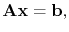(4.10)

where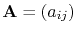is called stiffness matrix, given by the elements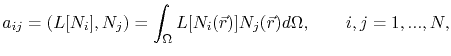(4.11)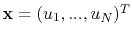is the vector of unknown coefficients, and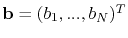is the load vector, given by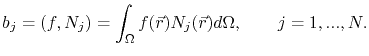(4.12)Next: 4.1.2 Assembly Up: 4.1 The Finite Element Previous: 4.1 The Finite Element

R. L. de Orio: Electromigration Modeling and Simulation